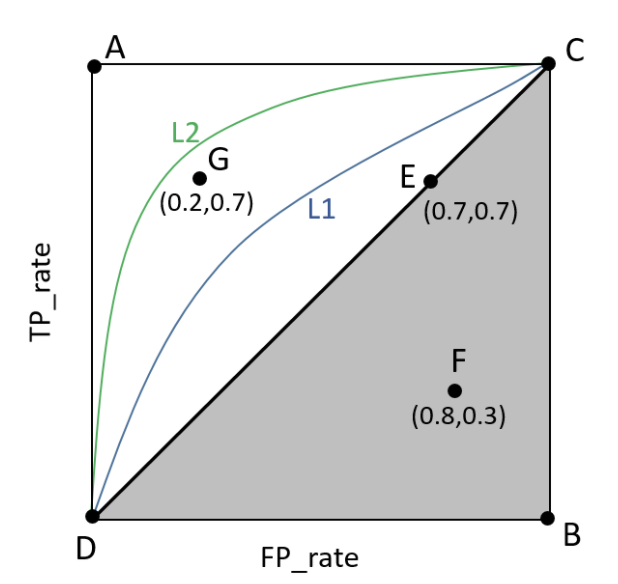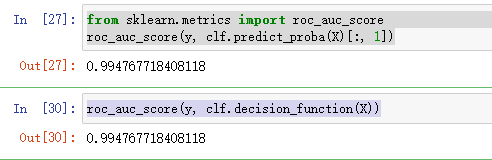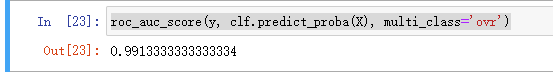• metrics.roc_auc_score
千次阅读
2022-03-10 17:10:34

# 二分类，sklearn包roc_curve计算曲线下面积

// 分割结果，单张图片AUC计算


必须保证y_true（gt）是二值、整型的，y_test（out_net）是网络输出结果，float数据，表示网络将像素分为0/1类的对应概率。

// code
from sklearn.metrics import roc_curve, auc
out_s = out_s.squeeze(0).detach().numpy()
gt_s = gt_s.squeeze(0).cpu().numpy().astype(int)

out_roc = out_s.reshape(-1, 1)
gt_roc = gt_s.reshape(-1, 1)
fpr, tpr, _ = roc_curve(gt_roc, out_roc)  # 真实值是int，行数为样本数，列数为1
roc_auc = auc(fpr, tpr)sklearn 分类 机器学习
更多相关内容
• from sklearn.metrics import roc_auc_score roc_auc_score(y_true, y_score, *, average='macro', sample_weight=None, max_fpr=None, multi_class='raise', labels=None) 计算曲线ROC的面积 - Parameters...

### 引言

from sklearn.metrics import roc_auc_score

roc_auc_score(y_true, y_score, *, average='macro', sample_weight=None,
max_fpr=None, multi_class='raise', labels=None)
计算曲线ROC的面积

- Parameters(参数)
y_true : array-like of shape (n_samples,) or (n_samples, n_classes)
真实数据
二分类和多分类需要带有shape (n_samples)的标签，而多标签情况需要带有shape (n_samples, n_classes)
的二进制标签。

y_score : array-like of shape (n_samples,) or (n_samples, n_classes)
预测结果数据
1.在二分类的情况下，它对应于形状数组（n_samples，），可以提供概率估计和非阈值决策值
概率估计值对应于具有更大标签的类别的概率，即estimator.classes_ ，
因此是estimator.predict_proba（X，y）[:, 1]。
决策值对应于estimator.decision_function（X，y）的输出。
2.在多分类情况下，它对应于由predict_proba方法提供的概率估计的形状数组（n_samples，n_classes）
每个sample概率估计值为1；此外，每一个sample的概率估计值的顺序必须与y_true中标签的顺序相对应。
3.在多标签情况下，它对应于一个形状数组（n_samples，n_classes）。
概率估计由predict_proba方法提供，非阈值决策值由decision_function方法提供。

average : {‘micro’, ‘macro’, ‘samples’, ‘weighted’} or None, default=’macro’
当y_true是二进制时，这个参数将被忽略
'macro':简单地计算 binary metrics （二分指标）的平均值，赋予每个类别相同的权重
'micro':给每个 sample-class pair （样本类对）对 overall metric （总体指数）
（sample-class 权重的结果除外） 等同的贡献。除了对每个类别的 metric 进行求和之外，这
个总和构成每个类别度量的 dividends （除数）和 divisors （除数）计算一个整体商。 在
multilabel settings （多标签设置）中，Micro-averaging 可能是优先选择的，包括要忽略
majority class （多数类）的 multiclass classification （多类分类）
'weighted': 通过计算其在真实数据样本中的存在来对每个类的 score 进行加权的 binary metrics （二分指标）
的平均值来计算类不平衡。
'samples':仅适用于多标签问题。它不计算每个类别的 measure，而是计算评估数据中的每个样本
的真实和预测类别的 metric （指标），并返回 (sample_weight-weighted) 加权平均。
sample_weight :array-like of shape (n_samples,), default=None
样品权重

sample_weight : array-like of shape (n_samples,), default=None
如果不为None，则返回范围为[0，max_fpr]的标准化部分AUC
对于多分类情况，max_fpr应该等于None或1.0

multi_class:{‘raise’, ‘ovr’, ‘ovo’}, default=’raise’
仅用于多分类，默认值会引发错误，因此必须显式传递'ovr'或'ovo'
'ovr':一对多
'ovo':一对一
这两个概念想了解的参考为2.逻辑回归部分

labels : array-like of shape (n_classes,), default=None
仅用于多分类，标签列表索引了y_score中的类，如果为None，则使用y_true中标签的数字或字典顺序

- 返回
AUC值



### 官方案例

二分类情况

>>> from sklearn.datasets import load_breast_cancer
>>> from sklearn.linear_model import LogisticRegression
>>> from sklearn.metrics import roc_auc_score
>>> clf = LogisticRegression(solver="liblinear", random_state=0).fit(X, y)
>>> roc_auc_score(y, clf.predict_proba(X)[:, 1])
0.99...
>>> roc_auc_score(y, clf.decision_function(X))
0.99...


多分类情况

>>> from sklearn.datasets import load_iris
>>> clf = LogisticRegression(solver="liblinear").fit(X, y)
>>> roc_auc_score(y, clf.predict_proba(X), multi_class='ovr')
0.99...


多标签情况

>>> from sklearn.datasets import make_multilabel_classification
>>> from sklearn.multioutput import MultiOutputClassifier
>>> X, y = make_multilabel_classification(random_state=0)
>>> clf = MultiOutputClassifier(clf).fit(X, y)
>>> # get a list of n_output containing probability arrays of shape
>>> # (n_samples, n_classes)
>>> y_pred = clf.predict_proba(X)
>>> # extract the positive columns for each output
>>> y_pred = np.transpose([pred[:, 1] for pred in y_pred])
>>> roc_auc_score(y, y_pred, average=None)
array([0.82..., 0.86..., 0.94..., 0.85... , 0.94...])
>>> from sklearn.linear_model import RidgeClassifierCV
>>> clf = RidgeClassifierCV().fit(X, y)
>>> roc_auc_score(y, clf.decision_function(X), average=None)
array([0.81..., 0.84... , 0.93..., 0.87..., 0.94...])


### 案例

import numpy as np
# 模型评估
from sklearn import metrics

if __name__ == '__main__':
y = np.array([0, 0, 1, 1])
y_pred = np.array([0.1, 0.5, 0.3, 0.8])

# 返回三个数组结果分别是fpr(假正率),tpr(召回率),threshold(阈值)
# 参数为真实结果数据、预测结果数据（可以是标签数据也可以是概率值）
fpr, tpr, threshold = metrics.roc_curve(y, y_pred)
# 计算AUC的值
auc = metrics.auc(fpr, tpr)
print(auc)
print(metrics.roc_auc_score(y, y_pred))

0.75
0.75


当碰到多分类情况时，可以使用one-hot编码，在进行ravel()展平操作，来使用

auc = roc_auc_score(y_one_hot.ravel(), y_score.ravel())


如果对您有帮助，麻烦点赞关注，这真的对我很重要！！！如果需要互关，请评论留言！展开全文• （1）曲线与FP_rate轴围成的面积（记作AUC）越大，说明性能越好，即图上L2曲线对应的性能优于曲线L1对应的性能。即：曲线越靠近A点（左上方）性能越好，曲线越靠近B点（右下方）曲线性能越差。 （2）A点是最完美的...

# 1.auc的计算原理

常用评价指标文章中摘出来：纵坐标为真阳性率（True Positive Rate, TPR）： TPR = TP / P，其中P是真实正样本的个数，TP是P个正样本中被分类器预测为正样本的个数。横坐标为假阳性率（False Positive Rate, FPR）： FPR = FP / N ，N是真实负样本的个数，FP是N个负样本中被分类器预测为正样本的个数。

（1）曲线与FP_rate轴围成的面积（记作AUC）越大，说明性能越好，即图上L2曲线对应的性能优于曲线L1对应的性能。即：曲线越靠近A点（左上方）性能越好，曲线越靠近B点（右下方）曲线性能越差。

（2）A点是最完美的performance点，B处是性能最差点。

（3）位于C-D线上的点说明算法性能和random猜测是一样的–如C、D、E点。位于C-D之上（即曲线位于白色的三角形内）说明算法性能优于随机猜测–如G点，位于C-D之下（即曲线位于灰色的三角形内）说明算法性能差于随机猜测–如F点。

（4）虽然ROC曲线相比较于Precision和Recall等衡量指标更加合理，但是其在高不平衡数据条件下的的表现仍然过于理想，不能够很好的展示实际情况。

# 2.sklearn.metrics.roc_auc_score()的使用方法

用法：计算auc

sklearn.metrics.roc_auc_score(y_true, y_score, *, average='macro', sample_weight=None, max_fpr=None, multi_class='raise', labels=None)[source])

输入参数：

y_true：真实的标签。形状(n_samples，)或(n_samples, n_classes)。二分类的形状(n_samples，1)，而多标签情况的形状(n_samples, n_classes)。

y_score：目标分数。形状(n_samples，)或(n_samples, n_classes)。二分类情况形状(n_samples，1)，“分数必须是具有较大标签的类的分数”，通俗点理解:模型打分的第二列。举个例子:模型输入的得分是一个数组[0.98361117 0.01638886]，索引是其类别，这里“较大标签类的分数”，指的是索引为1的分数：0.01638886，也就是正例的预测得分。

average='macro'：二分类时，该参数可以忽略。用于多分类，' micro '：将标签指标矩阵的每个元素看作一个标签，计算全局的指标。' macro '：计算每个标签的指标，并找到它们的未加权平均值。这并没有考虑标签的不平衡。' weighted '：计算每个标签的指标，并找到它们的平均值，根据支持度(每个标签的真实实例的数量)进行加权。(多分类的问题在下一篇文章中解释)

sample_weight=None：样本权重。形状(n_samples，)，默认=无。

max_fpr=None：

multi_class='raise'：(多分类的问题在下一篇文章中解释)

labels=None：

输出：

auc：是一个float的值。

# 3.举个例子

## 3.1数据格式：

 id label pred_label pred_score model_predict_scores 1537 0 0 0.98361117 [0.98361117 0.01638886] 1548 0 0 0.9303047 [0.9303047  0.06969527] 1540 0 0 0.978964 [0.978964   0.02103605] 15525 1 1 0.9876039 [0.01239602 0.9876039 ]

## 3.2代码：

先将模型预测的结果保存到excel中，将预测结果保存为了string，导致了下面的处理稍微麻烦了一点:

# -*- encoding:utf-8 -*-
import requests, xlrd, re, xlwt, json
from collections import defaultdict
from sklearn import metrics
sheets = workbook.sheet_names()  # 获取工作簿中的所有表格
worksheet = workbook.sheet_by_name(sheets)  # 获取工作簿中所有表格中的的第一个表格
label = [] # 真实标签
pred = [] # 预测标签
score = [] #跟预测标签对应的模型打分
first = [] # 模型打分结果中类别0的概率，是一个n行 ，1列的数组
preds = []  # 模型的打分结果中类别1的概率，是一个n行 ，1列的数组
for i in range(0, 100):
value = worksheet.cell_value(i, 1)
value1 = worksheet.cell_value(i, 2)
label.append(int(value))
pred.append(int(value1))
score.append(float(worksheet.cell_value(i, 3)))
a = worksheet.cell_value(i, 4)
d = a.replace('[', '')
d = d.replace(']', '')
d = d.strip()
d = d.split(" ")
l = len(d)
print(' len ', l)
g = []
h = []
h.append(float(d))
g.append(float(d[l - 1]))
preds.append(g)
first.append(h)
rocauc = metrics.roc_auc_score(label, score)
print('--rocauc:', rocauc)
rocauc1 = metrics.roc_auc_score(label, preds)
print('--rocauc1:', rocauc1)
rocauc0 = metrics.roc_auc_score(label, first)
print('--rocauc0:', rocauc0)

if __name__ == '__main__':
calculate_auc(read_path)

输出结果:

--rocauc: 0.3817052512704686
--rocauc1: 0.9390175042348956
--rocauc0: 0.06098249576510448

分析:

第一个结果，是错误 的结果，这么计算没有意义，解释不通，用错了预测值 。

第二个结果是label为1的auc，这个值是大家平时说的 auc。

第三个结果是label为0的auc，平时不用，这里打印出来主要是 想验证一下，正负样本的auc和是不是1。经过验证，二者和为1。

参考：

展开全文算法 python
• from sklearn.metrics import roc_auc_score roc_auc_score(y_true, y_score, *, average='macro', sample_weight=None, max_fpr=None, multi_class='raise', labels=None) 计算曲线ROC的面积 - Parameters(参数) ...

参数说明

from sklearn.metrics import roc_auc_score

roc_auc_score(y_true, y_score, *, average='macro', sample_weight=None,
max_fpr=None, multi_class='raise', labels=None)
计算曲线ROC的面积

- Parameters(参数)
y_true : array-like of shape (n_samples,) or (n_samples, n_classes)
真实数据
二分类和多分类需要带有shape (n_samples)的标签，而多标签情况需要带有shape (n_samples, n_classes)
的二进制标签。

y_score : array-like of shape (n_samples,) or (n_samples, n_classes)
预测结果数据
1.在二分类的情况下，它对应于形状数组（n_samples，），可以提供概率估计和非阈值决策值
概率估计值对应于具有更大标签的类别的概率，即estimator.classes_ ，
因此是estimator.predict_proba（X，y）[:, 1]。
决策值对应于estimator.decision_function（X，y）的输出。
2.在多分类情况下，它对应于由predict_proba方法提供的概率估计的形状数组（n_samples，n_classes）
每个sample概率估计值为1；此外，每一个sample的概率估计值的顺序必须与y_true中标签的顺序相对应。
3.在多标签情况下，它对应于一个形状数组（n_samples，n_classes）。
概率估计由predict_proba方法提供，非阈值决策值由decision_function方法提供。

average : {‘micro’, ‘macro’, ‘samples’, ‘weighted’} or None, default=’macro’
当y_true是二进制时，这个参数将被忽略
'macro':简单地计算 binary metrics （二分指标）的平均值，赋予每个类别相同的权重
'micro':给每个 sample-class pair （样本类对）对 overall metric （总体指数）
（sample-class 权重的结果除外） 等同的贡献。除了对每个类别的 metric 进行求和之外，这
个总和构成每个类别度量的 dividends （除数）和 divisors （除数）计算一个整体商。 在
multilabel settings （多标签设置）中，Micro-averaging 可能是优先选择的，包括要忽略
majority class （多数类）的 multiclass classification （多类分类）
'weighted': 通过计算其在真实数据样本中的存在来对每个类的 score 进行加权的 binary metrics （二分指标）
的平均值来计算类不平衡。
'samples':仅适用于多标签问题。它不计算每个类别的 measure，而是计算评估数据中的每个样本
的真实和预测类别的 metric （指标），并返回 (sample_weight-weighted) 加权平均。
sample_weight :array-like of shape (n_samples,), default=None
样品权重

sample_weight : array-like of shape (n_samples,), default=None
如果不为None，则返回范围为[0，max_fpr]的标准化部分AUC
对于多分类情况，max_fpr应该等于None或1.0

multi_class:{‘raise’, ‘ovr’, ‘ovo’}, default=’raise’
仅用于多分类，默认值会引发错误，因此必须显式传递'ovr'或'ovo'
'ovr':一对多
'ovo':一对一
这两个概念想了解的参考为2.逻辑回归部分

labels : array-like of shape (n_classes,), default=None
仅用于多分类，标签列表索引了y_score中的类，如果为None，则使用y_true中标签的数字或字典顺序

- 返回
AUC值

获取auc的方式

二分类：

from sklearn.datasets import load_breast_cancer
from sklearn.linear_model import LogisticRegression
from sklearn.metrics import roc_auc_score
clf = LogisticRegression(solver="liblinear", random_state=0).fit(X, y)

from sklearn.metrics import roc_auc_score
#方式一
roc_auc_score(y, clf.predict_proba(X)[:, 1])
#方式二
roc_auc_score(y, clf.decision_function(X))多分类：

from sklearn.datasets import load_iris

clf = LogisticRegression(solver="liblinear").fit(X, y)
clf.predict_proba(X)

from sklearn.metrics import roc_auc_score
#multi_class='ovr'
roc_auc_score(y, clf.predict_proba(X), multi_class='ovr')https://blog.csdn.net/weixin_46649052/article/details/112384745

展开全文• 写在前面： sklearn计算auc(一):使用sklearn.metrics.roc_auc_score()计算二分类的auc 1.sklearn.metrics.roc_auc_score()计算多分类auc的用法 2.例子python
• sklearn.metrics.roc_auc_score(y_true, y_score, *, average='macro', sample_weight=None, max_fpr=None, multi_class='raise', labels=None) 二分类 y_true：样本的真实标签，形状（样本数，） y_score：预测为1...
• ROC（Receiver Operating Characteristic）曲线和AUC常被用来评价一个二值分类器（binary classifier）的优劣。 AUC（Area Under Curve）被定义为ROC曲线下的面积，显然这个面积的数值不会大于1。又由于ROC曲线一般...sklearn
• 以前使用roc_auc_score都是用model.predict()的预测结果，错了那么久。。。。。。 查看官网注释才知道应该用model.predict_proba()的预测结果。 做了个对比试验，分别使用model.predict() 和 model.predict_proba...
• roc_auc_score则是不需要求这两个值，直接将预测概率值和label输入到函数中便可以求得auc值，省略了求这两个值的步骤，由函数本身代求。 这是我找很久的解释博客，才弄明白。 希望能帮助到大家。 ...python 机器学习
• import numpy as np from sklearn import metrics import matplotlib.pyplot as plt ...fpr,tpr,thresholds=metrics.roc_curve(label,scores) print('FPR:',fpr) print('TPR:',tpr) print('thresholds:',threshojson vscode
• accuracy_score 分类准确率分数是指所有分类正确的百分比。分类准确率这一衡量分类器的标准比较...sklearn.metrics.accuracy_score(y_true, y_pred, normalize=True, sample_weight=None) normalize：默认值为True...分类
• AUC原理可看：https://editor.csdn.net/md/?articleId=104838433 >>> import numpy as np ... from sklearn import metrics >>> y = np.array([1, 1, 2, 2]) >>> scores = np.array(...
• 1、sklearn.model_selection.cross_val_score(estimator,X,y=None,groups=None,scoring=None,cv=None,n_jobs=None,verbose=0,fit_params=None,pre_dispatch='2*n_jobs',error_score=nan) （1）cv参数定义交叉验证...机器学习
• 在使用sklearn.metrics.roc_curve过程中可能会遇见以下两种问题： 1.混淆矩阵与ROC曲线严重不符 如你的混淆矩阵长这样（图左），而你的ROC曲线长这样（图右） 2.报错No positive samples in y_true ...python sklearn 图像处理
• 1、accuracy_score 分类准确率分数是指所有分类正确的百分比。分类准确率这一衡量...sklearn.metrics.accuracy_score(y_true, y_pred, normalize=True, sample_weight=None)normalize：默认值为True，返回正确分...
• 讲解了fpr,tpr,threshold=roc_curve(y_true,y_score) 阈值是y_score内的元素去重后加入一个‘最大值+1’的值降序排序后组成的数据,每一个元素作为阈值，数据类型是一维数组。比如:y_score=np.array([0, 1, 2,0,3,1])...机器学习
• from sklearn.metrics import roc_auc_score y_true = [0, 0, 1, 1, 1] y_score = [0.1, 0.2, 0.7, 0.8, 0.9] print(roc_auc_score(y_true, y_score)) y_score = [0.7, 0.8, 0.9, 0.1, 0.2] print(roc_auc_score(y...
• ROCAUC计算， sklearn.metrics roc_curve、precision_recall_curve、aucroc_auc_score，多标签分类ROC曲线绘制python sklearn
• ## sklearn：auc、roc_curve、roc_auc_score

万次阅读 多人点赞 2018-10-25 19:23:04
sklearn.metrics.auc 作用：计算AUC(Area Under the Curve) metrics.roc_curve 作用：计算 ROC(Receiver operating characteristic) 注意: this implementation is restricted to the binary classification task ...
• >from sklearn.metrics import roc_auc_score >> >y_true = np.array([ 0 , 0 , 1 , 1 ]) >> >y_scores = np.array([ 0 . 1 , 0 . 4 , 0 . 35 , 0 . 8 ]) >> >roc_auc_score(y_true, y_scores) 0 . 75 ...
• 首先，你的数据不管是库自带的如： from sklearn.datasets import load_breast_cancer...X = data.drop("score", axis=1).values Y = data["score"].values 都要注意保证你的数据都是numpy类型的 对于二分类 直接用 Y
• 首先，roc_auc_score函数需要...sklearn.metrics.roc_auc_score(y_true, y_score, average=’macro’, sample_weight=None)Note: this implementation is restricted to the binary classification task or multilab...
• sklearn.metrics.auc只会计算label=1的auc，而tf.keras.metrics.AUC在多分类情况下，会将每一类的输出flattened，视作一列计算（每个样本对于每一类的数据均会被视为一个点），在样本不均衡的情况下，会得到较高的...深度学习 人工智能 算法
• 一、明白ROC的原理 ...深度学习对猫狗分类做ROC曲线时，使用from sklearn.metrics import roc_curve, auc是可以画出两条ROC曲线的。一条是猫，另一条是狗的ROC，最后取做平均。 二、from sklearn.metrics impo.
• from sklearn.metrics import accuracy_score,f1_score,roc_auc_score,recall_score,precision_score,confusion_matrix from sklearn.metrics import accuracy_score,f1_score,roc_auc_score,recall_score,...sklearn 召回率
• 关于运行roc_auc_score(y_true, y_scores)报错：ValueError: multiclass format is not supported，原因在于ROC只能用于二分类问题，所以，对于多分类问题，我们不用ROC曲线去评估，可以选用accuracy_score进行评估 ...
•   了解一个函数首先看这个函数的功能，也就是这个函数的参数有哪些，这个函数的返回值是哪些。...accuracy_score（）函数 1、函数功能 分类正确率分数，函数返回一个分数，这个分数或是正确的比.python 机器学习 人工智能...